The attenuation in a uniform differential pair has two root causes: conductor loss and dielectric loss. By understanding how design decisions affect these two fundamental root causes, we can develop a few simple guiding principles which point us in the right directions to reduce the attenuation of a channel. These are the directions to follow when loss is important. In some cases, increasing the differential impedance will decrease loss, and in some cases it will increase the loss. Read on to see why.

### Attenuation, Insertion Loss and SDD21

If we send a sine wave signal into a well matched, uniform differential channel without cross talk from other channels, the amplitude will decrease exponentially as it travels down the channel. This is true of 100% of all homogenous channels. This amplitude drop-off down an interconnect is shown in Figure 1.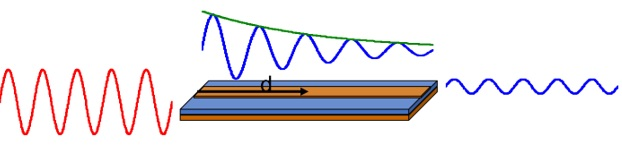Figure 1.  The amplitude of the signal decreases as it propagates down the interconnect.

The amplitude at some distance down the line is described by: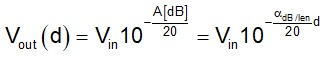Where

Vout(d) is the voltage amplitude some distance down the interconnect

Vin is the initial amplitude into the trace

A[dB] is the attenuation, in dB of the interconnect to the distance, d

α[dB/in] is the attenuation per length of the interconnect

d is the distance down the line

This is where the definition of attenuation comes from. It is the positive part of the exponent, in dB. The negative sign in the exponent is explicitly added to make the amplitude decrease with higher attenuation. Attenuation is positive. A larger value of attenuation in a channel means there is more signal loss and a smaller amplitude at the receiver, RX.

The attenuation  of all homogenous channels will increase linearly with the length of the channel. It is the exponent which increases linearly with distance.

We can generate another figure of merit, the attenuation per length, in dB/inch, which characterizes the channel. Attenuation per length is positive. A larger attenuation per length means there is more loss in the channel and less signal at the receiver. We use this term as a figure of merit to compare different channels, independent of their length.

The attenuation per length is inherently frequency dependent due to the two mechanisms: conductor loss and dielectric loss. The conductor loss increases with the square root of frequency due to current constriction from the skin depth effect. The dielectric loss increases directly with frequency due to the faster motion of the dipoles.

In a well-matched channel with no cross talk, SDD21, the transmission coefficient is also related to the attenuation: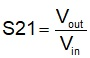And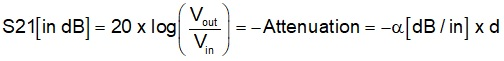Other than the minus sign, the SDD21 is exactly the same as the attenuation in the channel. The minus sign is where the confusion arises. When the attenuation of a channel increases, less signal arrives at the receiver, and the transmission coefficient, SDD21, decreases. It is appropriate to describe a more lossy channel as higher attenuation and a lower SDD21.

As an industry, we are in the habit of calling SDD21 the differential insertion loss, using the RF definition of insertion loss, which is |SDD21[dB]|. In reality, insertion loss is -SDD21[dB]. If the attenuation in a channel increases, SDD21[dB] decreases, but the insertion loss increases.

But, when we plot SDD21[dB], we often correctly plot it as a negative number, but incorrectly label the axis as insertion loss, which is supposed to be a positive number in dB. Can it get any more confusing? I confess I am more guilty than most of spreading this confusion.

If we use the RF definition of insertion loss, then higher loss in a channel means higher insertion loss. When we plot SDD21[dB], and label it as insertion loss, we just have to be aware we are incorrectly labeling the axis as insertion loss, when it should be labeled as the negative of the insertion loss.

The Root Cause of Insertion Loss

The insertion loss, |SDD21[dB]| will increase directly proportional to the distance traveled down the transmission line. The frequency dependence of insertion loss, in dB, comes from the frequency dependence of the conductor loss and the dielectric loss.

Using a simple model for a lossy line  and the “low loss” approximation, which basically means the dissipation factor is less than 0.05, the SDD21[dB] can be approximated as: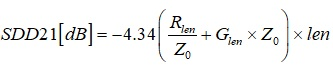Where

SDD21 = the differential transmission coefficient in dB

Rlen = the resistance per length of the differential pair

Z0 = the differential characteristic impedance of the pair

Glen = the conductance per length of the differential pair

len = the length of the differential pair

The first observation is, if you want to keep the transmission coefficient, SDD21, large, you want to use a short length. While it is rarely an option, always try to reduce the length of all lossy interconnections.

This relationship separates the conductor loss in the first term, and the dielectric loss in the second term. These two loss-mechanism are mostly independent.

Knobs to Reduce Dielectric Loss Term

There is an important connection between the conductance per length and the characteristic impedance. The same geometrical features that affect the conductance per length affect the characteristic impedance inversely. This means that the product of the two terms cancels out the geometry terms.

The dielectric loss term can be simplified as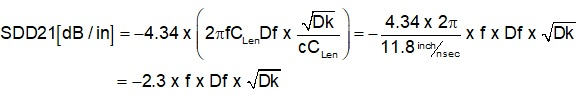This says that the only terms that affect SDD21 from dielectric loss are the dissipation factor, Df, and as a second order term, the dielectric constant, Dk. The transmission line physical design, such as dielectric thickness, line width, or even characteristic impedance have no effect on the insertion loss from the dielectric loss mechanism.

The frequency dependence of the SDD21 term from dielectric loss is due to the explicit frequency term created by the higher leakage current from the faster rotation of dipoles as the frequency increases.

If you want to reduce the SDD21 due to the dielectric, the only knob to tweak is a lower dissipation factor. A lower Dk will help, but it is a square root dependence, and there is not much range from which to choose.

Knobs to Adjust to Reduce Conductor Loss

When a low loss dielectric is used, such as Megtron 6 or 7 or even the new Rogers RO1200, the channel losses are dominated by conductor loss.

How do we reduce conductor loss? Assuming you are using the smoothest copper foil you can afford , the relationship above points out there are only two knobs to reduce copper loss: reduce the resistance per length or increase the differential impedance.

The only knob to adjust to reduce the resistance per length is to increase the line width of the trace. Wider lines will have lower resistance per length.

But, of course, the differential impedance will be affected by the line width as well. Generally, if only the line width increases, the differential impedance would decrease. Increasing the line width will decrease both the numerator and the denominator. Which one wins?

Here lies the complication. Depending on the impact of line width on resistance per length and differential impedance, the net impact is that a wider line might not reduce the insertion loss very much. This is where a careful analysis is important. A 2D field solver is a great tool to use as a virtual prototype to explore these tradeoffs.

Why is it that a higher differential impedance may have a lower loss than a lower differential impedance? If the differential impedance is increased without decreasing the line width, such as by using a thicker dielectric, the attenuation will decrease directly proportional to the differential impedance increase.

In going from 85 Ohms to 100 Ohms, at constant line width, the differential impedance increases by 18% and the insertion loss, if dominated by conductor loss, would decrease by as much as 18%.

If we engineer a wider line by keeping the differential impedance constant, the insertion loss will decrease proportional to the line width.

Here is how we achieve a wider line at constant differential impedance:

1. Use as thick dielectric layers as practical

2. Use as low a Dk between the signal and return planes as practical

3. Use a loosely coupled differential pair. (This allows a wider line than tightly coupled.)

4. Use as high a differential impedance as practical

5. Use the smoothest copper you can afford

When reducing loss is the main driving force in a design, these should be the starting place guidelines to engineer the lowest possible conductor loss.

What happens to the loss when only the line width is increased?

In the special case of a loosely coupled stripline differential pair, with 5 mil wide traces, engineered for 100 Ohms, we can use a 2D field solver to evaluate what impact there is on loss by increasing the line width.

The wider line will reduce the resistance, but it will also reduce the differential impedance. What happens to the ratio? In this example, we will look at a frequency of 14 GHz, the Nyquist for a 28 Gbps NRZ signal or 56 Gbps PAM4 signal.

Figure 2 shows the nominal starting place for the stackup design for this stripline.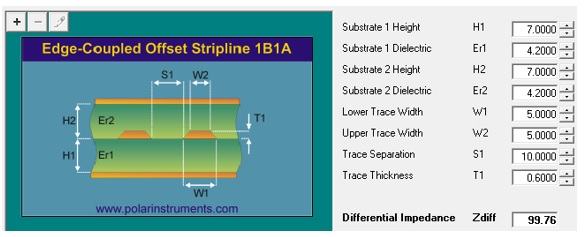Figure 2.  Set up of the Polar Instruments SI9000 2D field solver to explore design space for the special case of a 100 Ohm differential pair.

In this example, the dielectric loss is turned off, and we assume we are using very smooth copper. The insertion loss per inch from just the conductor loss is 0.404 dB/inch at 14 GHz. This means for a channel 20 inches long, the insertion loss at 14 GHz from the conductor loss is 0.404 x 20 = 8.1 dB and SDD21 is -8.1 dB. Rough copper and dielectric loss could increase this by a factor of 2-4.

If the only term to increase is the line width, the differential impedance will decrease. What happens to the insertion loss? By tweaking the line width of both traces, we calculate both the differential impedance and the insertion loss per inch at 14 GHz. Figure 3 is the plot of the differential impedance and the transmission coefficient, SDD21, just from smooth copper loss as the line width increases.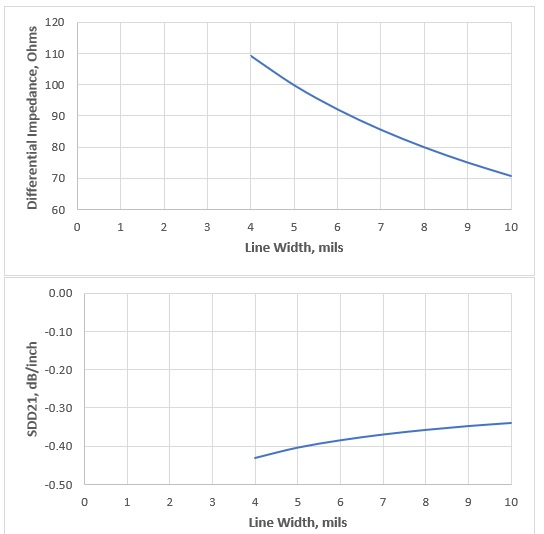Figure 3.  The impact on differential impedance (top) from just increasing the linewidth and on the transmission coefficient(bottom).

The insertion loss does get smaller as the line width gets wider, but the impact is not as large as expected from just a line width increase.

For example, with a 5 mil wide trace at 100 Ohm differential impedance, the insertion loss is 0.404 dB/inch at 14 GHz. Just increasing the line width to 7 mils decreases the differential impedance to 85 Ohms and the insertion loss increases to 0.37 dB/inch.

The line width increased by 40%, but the insertion loss increased by only 8%. This is why, to achieve the most reduction in insertion loss, increase the line width while NOT changing the differential impedance.

In this example, we reduced the differential impedance and the insertion loss decreased. But, if we had kept the line width constant and decreased the differential impedance, the insertion loss would have increased from conductor loss. At the same time, the dielectric loss would have stayed the same, independent of differential impedance, since it is not about the geometry, only about the material properties.

### Conclusion

When exploring design space for a differential channel, it is important to consider the root cause of the sources of loss and how both design and materials options play together. Reducing conductor loss is not just about increasing the line width, it is also about decreasing the differential impedance.

If all we do is decrease the differential impedance, the insertion loss may increase if the line width is held constant, or decrease, if the line width is changed to adjust the differential impedance. The details matter. These tradeoffs can only be explored with a 2D field solver.

### References

1. Bogatin, Eric. Signal and Power Integrity - Simplified (Signal Integrity Library) 3rd Edition, Prentice Hall, 2017.

2. Bogatin, Eric. “The Quest for Smoother Copper May Have Reached Its Limit,” Signal Integrity Journal, February 25, 2020.